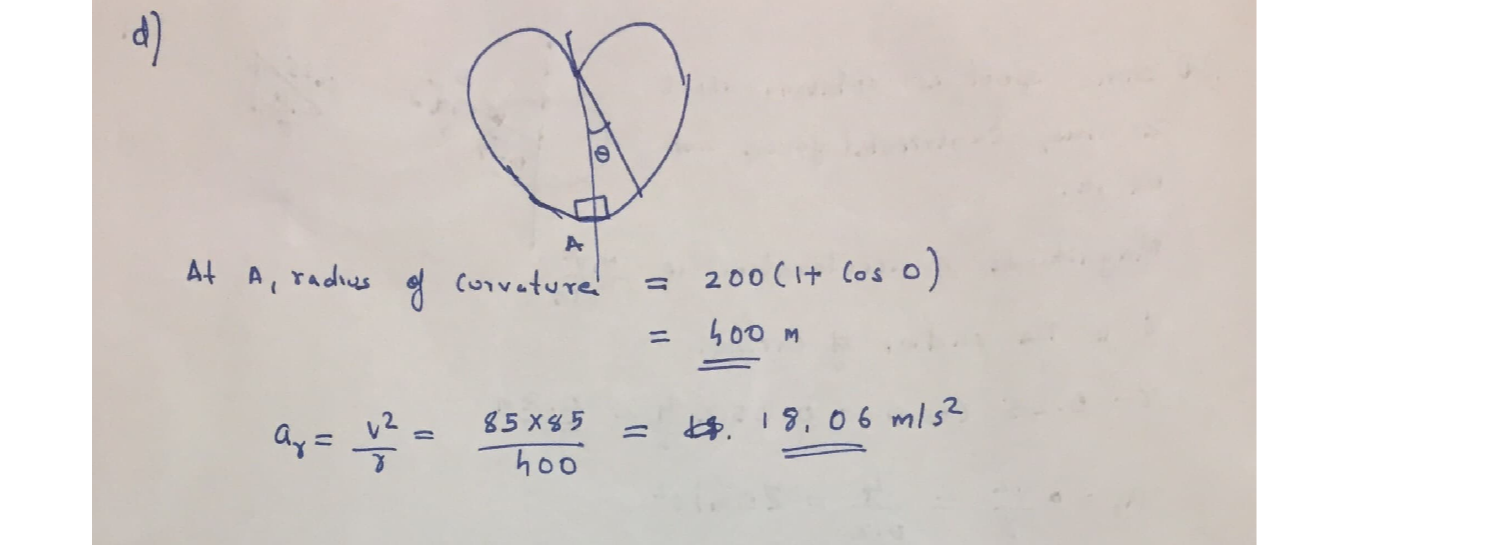Question

Dynamics

Problem 1: Find the magnitude of the acceleration of the particle in each of the following cases, Also, show the direction of the acceleration vector via an arrow in the figure.

(a) Spool S is sitting on the inclined rod at a distance 0.25m from A. The inclined rod is rotating about z axis, such that the speed of S is 2m/s and is constant. Note that the path traced by S is a circle as shown.

(c) A girl is sitting on a horizontal platform at a distance r = 5m from the center of the platform. The speed of the girl is 2m/s but the speed is decreasing at the rate of 0.8 m/s?.

(b) Spool S is sitting on the inclined rod at a distance 0.25m from A. Theinclined rod is rotating about z axis with a constant angular velocityof 2 rad/s.

200(1+ cos 0) with a(d) A pilot is making a loop on the curve r =constant speed of 85 m/s and is currently at point A (0 = 0).Verified### Question 45555Dynamics

*15-40. The boy jumps off the flat car at A with a velocity of v = 4 ft/s relative to the car as shown. If he lands on the second flat car B, determine the final speed of both cars after the motion. Each car has a weight of 80 lb. The boy's weight is 60 lb. Both cars are originally at rest. Neglect the mass of the car's wheels

### Question 45554Dynamics

15-57. The 10-kg block is held at rest on the smooth inclined plane by the stop block at A. If the 10-g bullet is traveling at 300 m/s when it becomes embedded in the10-kg block, determine the distance the block will slide up along the plane before momentarily stopping.

### Question 45553Dynamics

15-54. Solve Prob. 15-53 if the coefficient of kinetic friction between A and B is H = 0.3. Neglect friction between block B and the horizontal plane

### Question 45552Dynamics

15-15. The motor, M, pulls on the cable with a force F=(10f + 300) N, where r is in seconds. If the 100 kg crate's originally at rest at t=0, determine its speed when t=4 s Neglect the mass of the cable and pulleys. Hint: First find the time needed to begin lifting the crate.

### Question 45551Dynamics

15-22. The thrust on the 4-Mg rocket sled is shown in the graph. Determine the sleds maximum velocity and the distance the sled travels when t 35 s. Neglect friction.

### Question 45550Dynamics

15-10. The 50-kg crate is pulled by the constant force P. Ifthe crate starts from rest and achieves a speed of 10 m/s in5 s, determine the magnitude of P. The coefficient of kinetic friction between the crate and the ground is Hi =0.2.

### Question 45549Dynamics

*15-8. The automobile has a weight of 2700 lb and is-traveling forward at 4 ft/s when it crashes into the wall. Ifthe impact occurs in 0.06 s, determine the average impulsive force acting on the car. Assume the brakes are not applied. If the coefficient of kinetic friction between the wheels and the pavement is H = 0.3, calculate the impulsive force on the wall if the brakes were applied during the crash.The brakes are applied to all four wheels so that all the wheels slip.

### Question 45467Dynamics

As a part of a robotics competition, a robotic arm with a rigid open hand at C is to be designed so that the hand catches an egg without breaking it. The egg is released from rest at t = 0 from point A. The arm, initially at rest in the position shown, starts moving when the egg is released. The hand must catch the egg without any impact with the egg. This can be done by specifying that the hand and the egg must be at the same position at the same time with identical velocities. A student proposes to do this using a constant value of 0 for which (after a fair bit of work) it is found that the arm catches the egg at /= 0.4391 s forÖ = -13.27 rad/s2. Using these values of t and Ö, determine the acceleration of both the hand and the egg at the time of catch.Then, explain whether or not using a constant value of 0, as has been proposed, is an acceptable strategy.

### Question 45466Dynamics

A crate A is being pulled up an inclined ramp by a winch retracting the cord at a constant rate vo = 2 ft/s. Letting h =1.5 ft,determine the speed of the crate when d = 4 ft.

### Question 45463Dynamics

At the instant shown, VB = 5 î m/s. If theta = 25°, determine the speed of A relative to B in order for A to travel only in the vertical direction while sliding over B.

### Submit query